NCERT Questions

Class 9
Chapter 8 Class 9 - Motion

## (a) Find how far does the car travel in the first 4 seconds. Shade the area on the graph that represents the distance travelled by the car during the period. (b) Which part of the graph represents uniform motion of the car?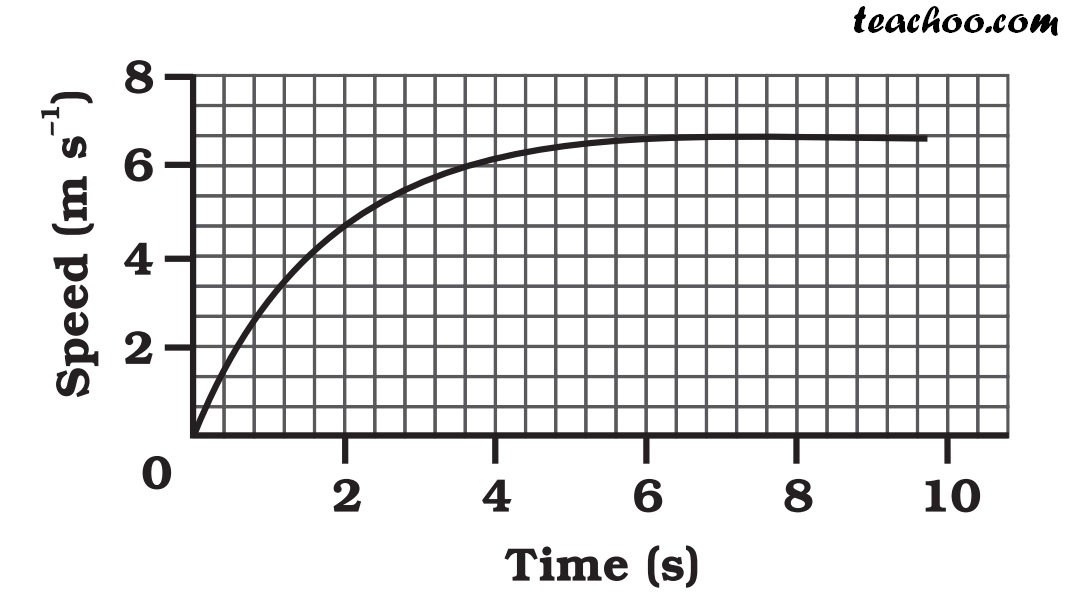(b)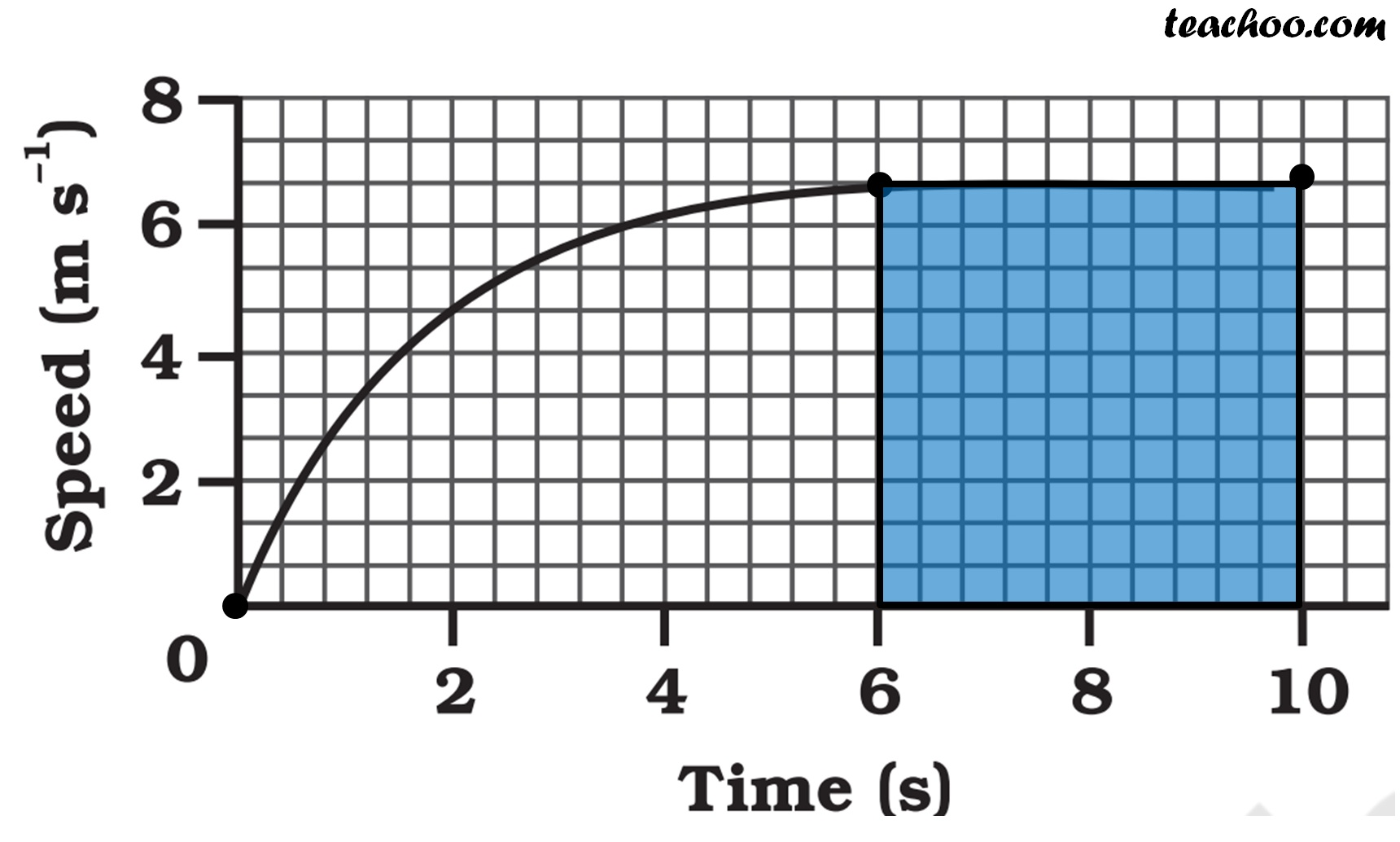The part of the graph between time 6s to 10s represents uniform motion of the car

(a)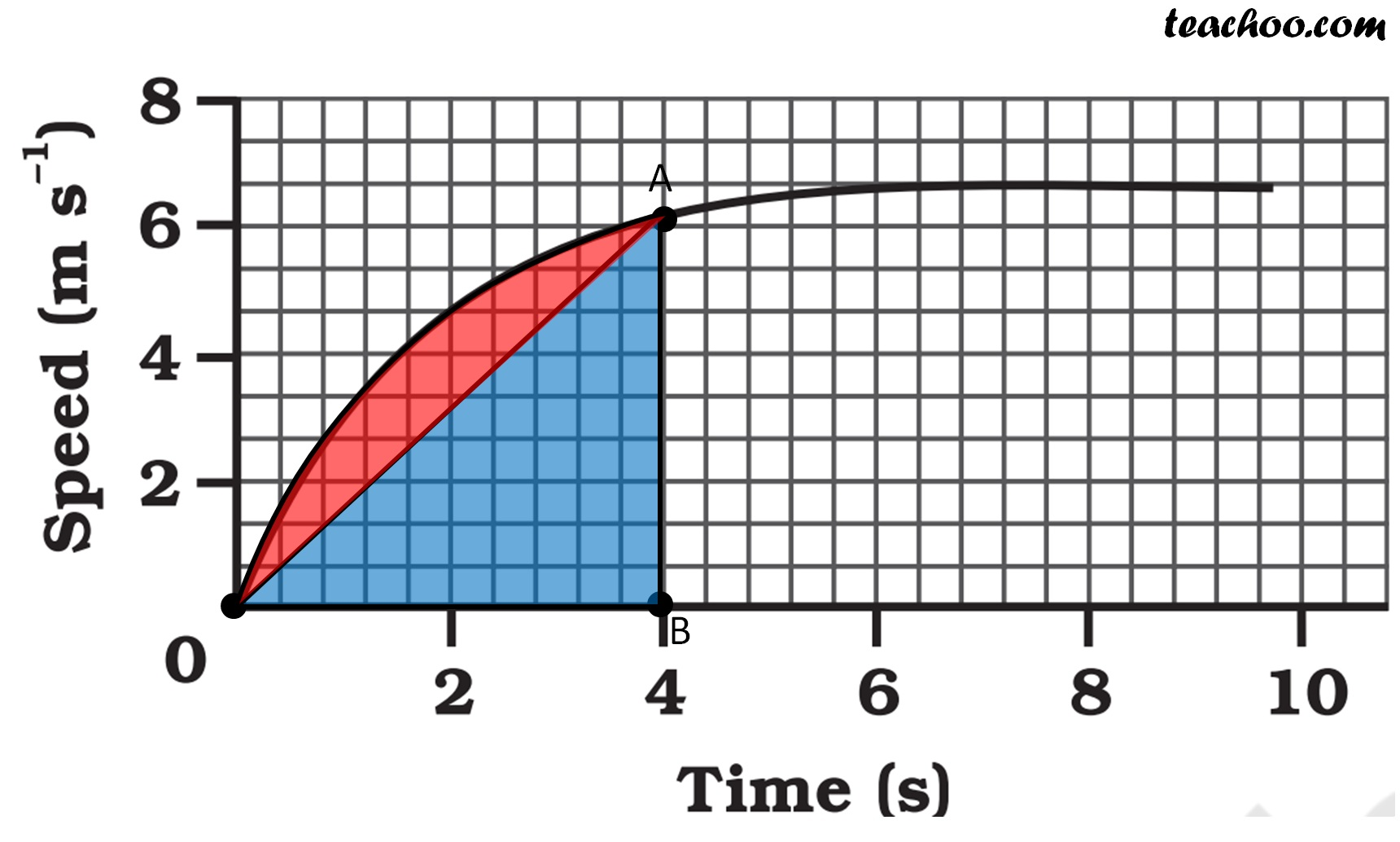In a velocity − time graph,

Distance = Area of v-t Graph

Here, we find approximate area using Area of Triangle

Distance = Area Δ OAB

= 1/2 × b × h

= 1/2 × 4 × 6

= 12 m

But, this is an inaccurate method to find Area, since the red portion is

not covered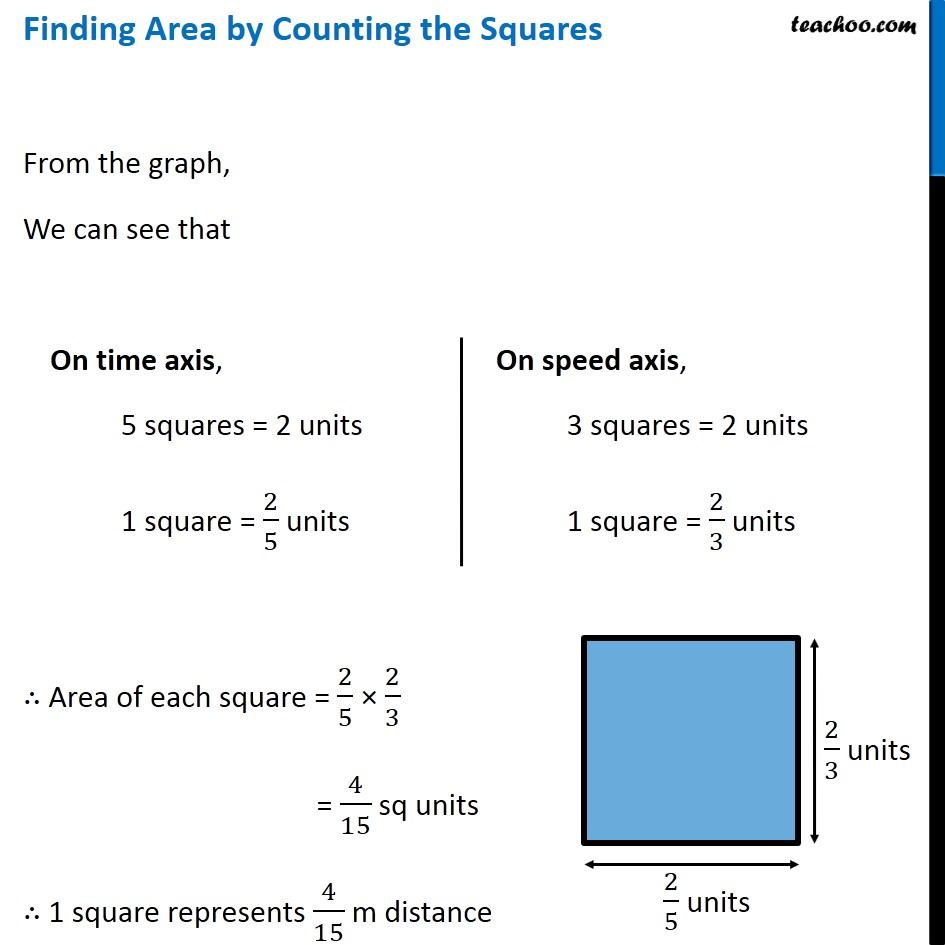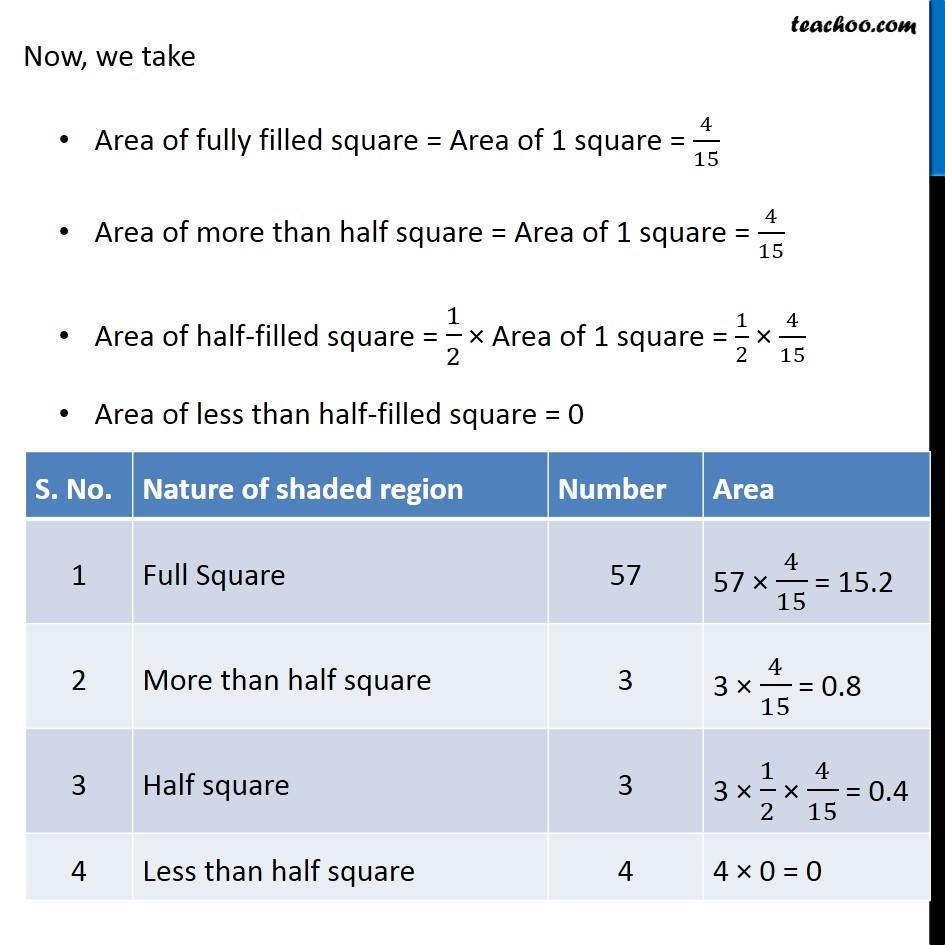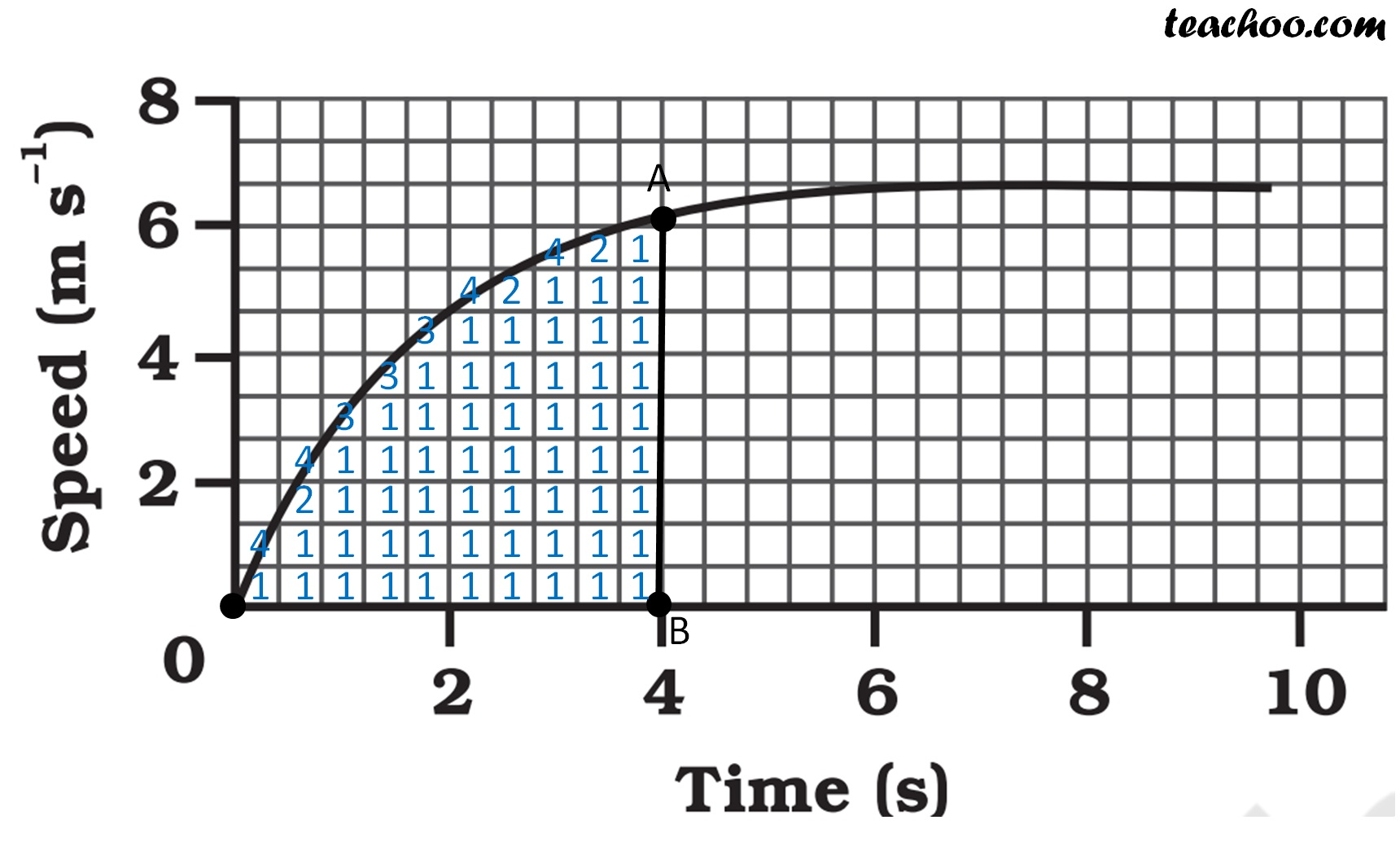∴ Total area = 15.2 + 0.8 + 0.4 + 0

= 16.4 sq units

Area under v − t graph gives distance

Hence, the distance covered by the car in 0−4 s = 16.4 m

Learn in your speed, with individual attention - Teachoo Maths 1-on-1 Class

### Transcript

Finding Area by Counting the Squares From the graph, We can see that On time axis, 5 squares = 2 units 1 square = 2/5 units On speed axis, 3 squares = 2 units 1 square = 2/3 units ∴ Area of each square = 2/5 × 2/3 = 4/15 sq units ∴ 1 square represents 4/15 m distance Now, we take Area of fully filled square = Area of 1 square = 4/15 Area of more than half square = Area of 1 square = 4/15 Area of half-filled square = 1/2 × Area of 1 square = 1/2 × 4/15 Area of less than half-filled square = 0 Area of less than half square = 0